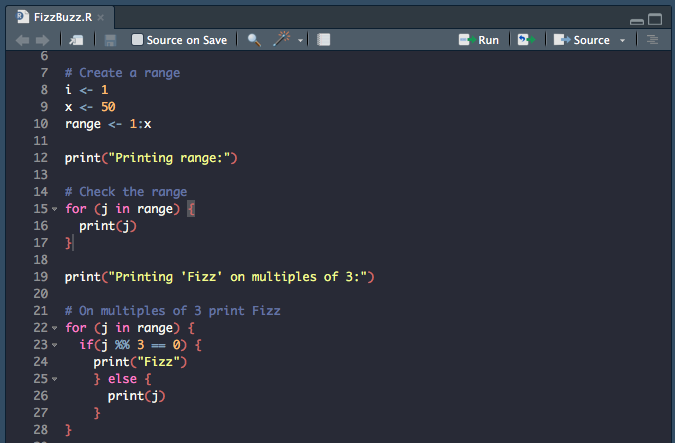# Fizz Buzz for R and Python — An Interview Question for Data Scientists

If you’re going to be applying for data science roles, eventually an interviewer is going to ask about your coding skills.Sample R input for the coding exercise

A classic question to ask for any interview is the FizzBuzz question.

You’ll be asked to write a simple program that prints the numbers from 1 to N. But for multiples of three print “Fizz” instead of the number. For the multiples of five print “Buzz”. And, for multiples of both three and five print, “FizzBuzz”.

Simple as. And I’m sure a data scientist who can code a neural net can make a simple program like this.

Maybe? Maybe .. not?

But you might as well make sure you know this so you don’t show up to the interview empty-handed.

The repo here has both sets of code in R and Python, but the examples here are only going to be in R. I’ll be going over how to do this exercise with `for` and `while` loops.

There are many ways to do this exercise and don’t take this as FizzBuzz dogma.

The first thing we need to do is create our `index` variable `i` and upper limit `x` of the `range` we’re going to be printing out. We’ll be using `i` for the `while` loops, but not the `for` loops.

`i <- 1x <- 50range <- 1:x`

Let’s print out our inclusive range, from 1 to 50.

`for (j in range) {  print(j)}`
`>> 1 2 3 4... 47 48 49 50`

Looks like our range is correct. Let’s try printing `Fizz` using a `for` loop, on multiples of three. Also notice, we’re using the `%%` operator which is the `mod` operator. It returns the remainder of a division.

`x %% y <=> remainder of x divided by y  <=> (x mod y)`
`7 %% 3 = 17 %% 4 = 38 %% 6 = 210 %% 5 = 012 %% 3 = 0`

If a number is a multiple of another number, it will return zero. So for multiples of 3, we’re looking at the numbers 3, 6, 9, 12, etc.

Now we can move on to `Fizz`.

`print("Printing 'Fizz' on multiples of 3:")`
`for (j in range) {  if(j %% 3 == 0) {    print("Fizz")`
`    } else {      print(j)`
`    }}`
`>> 1 2 "Fizz" 4 5 "Fizz"... "Fizz" 46 47 "Fizz" 49 50`

Looks like that is working as expected. Now let’s try the same thing, but using a `for` loop. Notice the `next` flow control in the while loop. When that statement is reached, the while loop returns to the beginning and executes again.

`print("Printing 'Fizz' on multiples of 3. Using a while loop:")`
`while (i <= x) {  if(i %% 3 == 0) {    print('Fizz')    i <- i + 1    next    }  print(i)  i <- i + 1`
`}`
`# Reset indexi <- 1`
`>> 1 2 "Fizz" 4 5 "Fizz"... "Fizz" 46 47 "Fizz" 49 50`

Now, on to `Buzz`.

`print("Printing 'Buzz' on multiples of 5:")`
`for (j in range) {  if(j %% 5 == 0) {    print('Buzz')    } else {    print(j)  }}`
`>> 1 2 3 4 "Buzz"... "Buzz" 46 47 48 49 "Buzz"`

Here comes the fun part: printing `FizzBuzz`. And, there is a trick to getting it right. You want to have the if statement check if `j` is a multiple of 3 and 5 before it checks for them individually. If it is a multiple of 3 and 5, the loop will reset and go to the next value in the range, if not it will try 5 and then 3. And, finally, if none are multiples of `j` it will print `j` and go on to the next value in the range until the end.

`for (j in range) {    if(j %% 3 ==  0 && j %% 5 ==  0) {    print("FizzBuzz")    } else if (j %% 5 == 0) {    print("Buzz")    } else if (j %% 3 ==  0){   print("Fizz")    } else {    print(j)`
`  }}`
`>> 1 2 "Fizz" 4 "Buzz"... 11 "Fizz" 13 14 "FizzBuzz" 16 17 "Fizz"... "FizzBuzz" 46 47 "Fizz" 49 "Buzz"`

Finally, let’s do the same `FizzBuzz` with a `while` loop. Not much has changed, except we’ll be using the `next` flow control again.

`while (i <= x) {    if (i %% 3 == 0 && i %% 5 == 0) {    print("FizzBuzz")    i <- i + 1    next  }    if (i %% 5 == 0) {    print('Buzz')    i <- i + 1    next  }    if (i %% 3 == 0) {    print('Fizz')    i <- i + 1    next  }    print(i)  i <- i + 1}`
`>> 1 2 "Fizz" 4 "Buzz"... 11 "Fizz" 13 14 "FizzBuzz" 16 17 "Fizz"... "FizzBuzz" 46 47 "Fizz" 49 "Buzz"`

That’s it. This is fairly straightforward, once you get an understanding of the syntax and logic. And, now you’re ready for that easy interview question which will trip you up. If you want to see how it is done with python, check out the repo here.

Cheers.

Additional Watching:

https://www.youtube.com/watch?v=QPZ0pIK_wsc&vl=en